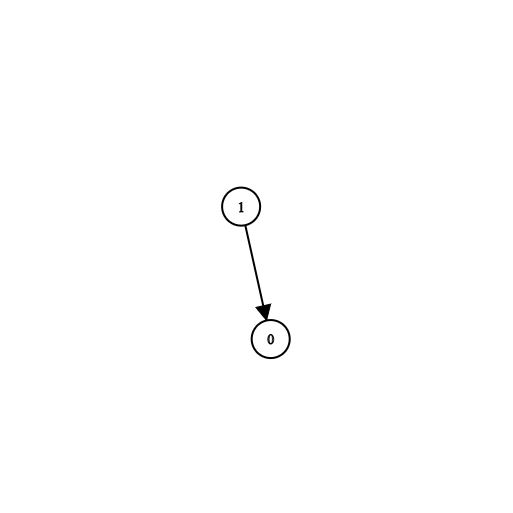Formatted question description: https://leetcode.ca/all/1462.html

# 1462. Course Schedule IV (Medium)

There are a total of n courses you have to take, labeled from 0 to n-1.

Some courses may have direct prerequisites, for example, to take course 0 you have first to take course 1, which is expressed as a pair: [1,0]

Given the total number of courses n, a list of direct prerequisite pairs and a list of queries pairs.

You should answer for each queries[i] whether the course queries[i] is a prerequisite of the course queries[i] or not.

Return a list of boolean, the answers to the given queries.

Please note that if course a is a prerequisite of course b and course b is a prerequisite of course c, then, course a is a prerequisite of course c.

Example 1:Input: n = 2, prerequisites = [[1,0]], queries = [[0,1],[1,0]]
Output: [false,true]
Explanation: course 0 is not a prerequisite of course 1 but the opposite is true.


Example 2:

Input: n = 2, prerequisites = [], queries = [[1,0],[0,1]]
Output: [false,false]
Explanation: There are no prerequisites and each course is independent.


Example 3:Input: n = 3, prerequisites = [[1,2],[1,0],[2,0]], queries = [[1,0],[1,2]]
Output: [true,true]


Example 4:

Input: n = 3, prerequisites = [[1,0],[2,0]], queries = [[0,1],[2,0]]
Output: [false,true]


Example 5:

Input: n = 5, prerequisites = [[0,1],[1,2],[2,3],[3,4]], queries = [[0,4],[4,0],[1,3],[3,0]]
Output: [true,false,true,false]


Constraints:

• 2 <= n <= 100
• 0 <= prerequisite.length <= (n * (n - 1) / 2)
• 0 <= prerequisite[i], prerequisite[i] < n
• prerequisite[i] != prerequisite[i]
• The prerequisites graph has no cycles.
• The prerequisites graph has no repeated edges.
• 1 <= queries.length <= 10^4
• queries[i] != queries[i]

Related Topics:
Graph

## Solution 1. DFS

We can first build a directed graph given the prerequisite. Then for each query, we DFS to check we can reach q from q. To save computation, we can memoize the result of any from, to node pairs we’ve seen along the DFS process.

// OJ: https://leetcode.com/problems/course-schedule-iv/

// Time: O(V + E)
// Space: O(V^2)
class Solution {
vector<vector<int>> G, m;
bool dfs(int from, int to) {
if (m[from][to] != -1) return m[from][to];
bool ans = false;
for (int v : G[from]) {
if (dfs(v, to)) {
ans = true;
break;
}
}
return m[from][to] = ans;
}
public:
vector<bool> checkIfPrerequisite(int n, vector<vector<int>>& A, vector<vector<int>>& Q) {
G.assign(n, {});
m.assign(n, vector<int>(n, -1));
for (int i = 0; i < n; ++i) m[i][i] = 1;
for (auto &e : A) G[e].push_back(e);
vector<bool> ans;
for (auto &e : Q) ans.push_back(dfs(e, e));
return ans;
}
};


## Solution 2. Floyd-Warshall

We can use the Floyd-Warshall algorithm to fine all the shortest paths of any node pairs in a weighted graph. Here we can make simple adjustment to it to just store whether any node pairs are reachable.

// OJ: https://leetcode.com/problems/course-schedule-iv/

// Time: O(V^3)
// Space: O(V^2)
class Solution {
public:
vector<bool> checkIfPrerequisite(int n, vector<vector<int>>& A, vector<vector<int>>& Q) {
vector<vector<bool>> m(n, vector<bool>(n));
for (auto &e : A) m[e][e] = true;
for (int i = 0; i < n; ++i) {
for (int u = 0; u < n; ++u) {
for (int v = 0; v < n; ++v) {
m[u][v] = m[u][v] || (m[u][i] && m[i][v]);
}
}
}
vector<bool> ans;
for (auto &q : Q) ans.push_back(m[q][q]);
return ans;
}
};


Java

class Solution {
public List<Boolean> checkIfPrerequisite(int n, int[][] prerequisites, int[][] queries) {
Map<Integer, Set<Integer>> prerequisiteMap = new HashMap<Integer, Set<Integer>>();
for (int[] prerequisite : prerequisites) {
int course0 = prerequisite, course1 = prerequisite;
Set<Integer> set = prerequisiteMap.getOrDefault(course0, new HashSet<Integer>());
prerequisiteMap.put(course0, set);
}
List<Boolean> checkList = new ArrayList<Boolean>();
Map<Integer, Boolean> queryMap = new HashMap<Integer, Boolean>();
int queriesCount = queries.length;
for (int i = 0; i < queriesCount; i++) {
int[] query = queries[i];
boolean isPrerequisite = search(prerequisiteMap, queryMap, query, query, n);
}
return checkList;
}

public boolean search(Map<Integer, Set<Integer>> prerequisiteMap, Map<Integer, Boolean> queryMap, int course0, int course1, int n) {
int key = course0 * n + course1;
if (queryMap.containsKey(key))
return queryMap.get(key);
Set<Integer> set = prerequisiteMap.getOrDefault(course0, new HashSet<Integer>());
if (set.contains(course1)) {
queryMap.put(key, true);
return true;
} else {
for (int nextCourse : set) {
if (search(prerequisiteMap, queryMap, nextCourse, course1, n)) {
queryMap.put(key, true);
return true;
}
}
}
queryMap.put(key, false);
return false;
}
}